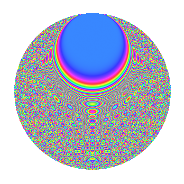# Properties

 Label 1575.2.csLevel 1575 Weight 2 Character orbit cs Rep. character $$\chi_{1575}(46,\cdot)$$ Character field $$\Q(\zeta_{15})$$ Dimension 784 Sturm bound 480

# Learn more about

## Defining parameters

 Level: $$N$$ = $$1575 = 3^{2} \cdot 5^{2} \cdot 7$$ Weight: $$k$$ = $$2$$ Character orbit: $$[\chi]$$ = 1575.cs (of order $$15$$ and degree $$8$$) Character conductor: $$\operatorname{cond}(\chi)$$ = $$175$$ Character field: $$\Q(\zeta_{15})$$ Sturm bound: $$480$$

## Dimensions

The following table gives the dimensions of various subspaces of $$M_{2}(1575, [\chi])$$.

Total New Old
Modular forms 1984 816 1168
Cusp forms 1856 784 1072
Eisenstein series 128 32 96

## Trace form

 $$784q$$ $$\mathstrut +\mathstrut 3q^{2}$$ $$\mathstrut +\mathstrut 93q^{4}$$ $$\mathstrut +\mathstrut 5q^{5}$$ $$\mathstrut -\mathstrut 14q^{7}$$ $$\mathstrut +\mathstrut 38q^{8}$$ $$\mathstrut +\mathstrut O(q^{10})$$ $$784q$$ $$\mathstrut +\mathstrut 3q^{2}$$ $$\mathstrut +\mathstrut 93q^{4}$$ $$\mathstrut +\mathstrut 5q^{5}$$ $$\mathstrut -\mathstrut 14q^{7}$$ $$\mathstrut +\mathstrut 38q^{8}$$ $$\mathstrut -\mathstrut 7q^{10}$$ $$\mathstrut -\mathstrut 12q^{13}$$ $$\mathstrut -\mathstrut 6q^{14}$$ $$\mathstrut +\mathstrut 93q^{16}$$ $$\mathstrut +\mathstrut 3q^{17}$$ $$\mathstrut -\mathstrut 11q^{19}$$ $$\mathstrut +\mathstrut 32q^{20}$$ $$\mathstrut +\mathstrut 12q^{22}$$ $$\mathstrut +\mathstrut 5q^{23}$$ $$\mathstrut -\mathstrut 7q^{25}$$ $$\mathstrut -\mathstrut 44q^{26}$$ $$\mathstrut -\mathstrut 38q^{28}$$ $$\mathstrut -\mathstrut 9q^{31}$$ $$\mathstrut +\mathstrut 40q^{32}$$ $$\mathstrut +\mathstrut 28q^{34}$$ $$\mathstrut -\mathstrut 8q^{35}$$ $$\mathstrut +\mathstrut 11q^{37}$$ $$\mathstrut +\mathstrut 10q^{38}$$ $$\mathstrut +\mathstrut q^{40}$$ $$\mathstrut -\mathstrut 2q^{41}$$ $$\mathstrut +\mathstrut 36q^{43}$$ $$\mathstrut +\mathstrut 23q^{44}$$ $$\mathstrut -\mathstrut 43q^{46}$$ $$\mathstrut +\mathstrut 5q^{47}$$ $$\mathstrut -\mathstrut 6q^{49}$$ $$\mathstrut +\mathstrut 82q^{50}$$ $$\mathstrut -\mathstrut 70q^{52}$$ $$\mathstrut +\mathstrut 23q^{53}$$ $$\mathstrut -\mathstrut 52q^{55}$$ $$\mathstrut +\mathstrut 60q^{56}$$ $$\mathstrut -\mathstrut 59q^{58}$$ $$\mathstrut +\mathstrut 15q^{59}$$ $$\mathstrut -\mathstrut 13q^{61}$$ $$\mathstrut +\mathstrut 92q^{62}$$ $$\mathstrut -\mathstrut 214q^{64}$$ $$\mathstrut -\mathstrut q^{65}$$ $$\mathstrut -\mathstrut 45q^{67}$$ $$\mathstrut +\mathstrut 122q^{68}$$ $$\mathstrut -\mathstrut 53q^{70}$$ $$\mathstrut +\mathstrut 10q^{71}$$ $$\mathstrut +\mathstrut 47q^{73}$$ $$\mathstrut +\mathstrut 22q^{74}$$ $$\mathstrut -\mathstrut 228q^{76}$$ $$\mathstrut -\mathstrut 9q^{77}$$ $$\mathstrut +\mathstrut q^{79}$$ $$\mathstrut +\mathstrut 70q^{80}$$ $$\mathstrut +\mathstrut 28q^{82}$$ $$\mathstrut +\mathstrut 26q^{83}$$ $$\mathstrut -\mathstrut 98q^{85}$$ $$\mathstrut +\mathstrut 41q^{86}$$ $$\mathstrut -\mathstrut 14q^{88}$$ $$\mathstrut +\mathstrut 42q^{89}$$ $$\mathstrut -\mathstrut 38q^{91}$$ $$\mathstrut +\mathstrut 154q^{92}$$ $$\mathstrut -\mathstrut 43q^{94}$$ $$\mathstrut +\mathstrut 57q^{95}$$ $$\mathstrut -\mathstrut 168q^{97}$$ $$\mathstrut -\mathstrut 106q^{98}$$ $$\mathstrut +\mathstrut O(q^{100})$$

## Decomposition of $$S_{2}^{\mathrm{new}}(1575, [\chi])$$ into irreducible Hecke orbits

The newforms in this space have not yet been added to the LMFDB.

## Decomposition of $$S_{2}^{\mathrm{old}}(1575, [\chi])$$ into lower level spaces

$$S_{2}^{\mathrm{old}}(1575, [\chi]) \cong$$ $$S_{2}^{\mathrm{new}}(175, [\chi])$$$$^{\oplus 3}$$$$\oplus$$$$S_{2}^{\mathrm{new}}(525, [\chi])$$$$^{\oplus 2}$$